## Journal of Biochemistry and Biotechnology

All submissions of the EM system will be redirected to Online Manuscript Submission System. Authors are requested to submit articles directly to Online Manuscript Submission System of respective journal.

Review Article - Journal of Biochemistry and Biotechnology (2021) Volume 4, Issue 4

## Modelling of index system of economic vitality during the COVID-19 epidemic

Shenglan Chu1, Jinming Cao2, Bin Zhao1*

1Department of Economics and Management, Hubei University of Technology, School of Economics and Management, Wuhan, Hubei, China

2Department of Information and Mathematics, Yangtze University, School of Information and Mathematics, Jingzhou, Hubei, China

Corresponding Author:
Bin Zhao
School of Economics and Management
Hubei University of Technology
Wuhan, Hubei, China
Tel: +8613028517572
E-mail: [email protected]

Accepted date: July 22, 2021

Citation: Chu S, Cao J, Zhao B. Modelling of index system of economic vitality during the COVID-19 epidemic. 2021;4(4):7-21.

Visit for more related articles at Journal of Biochemistry and Biotechnology

## Abstract

Economic vitality is an important indicator to measure the level and potential of economic development. The paper puts forward three social problems about economic vitality and establishes a model to solve them. We build panel data model to analyze the influencing factors of economic vitality. Based on the section data of Beijing, the VAR-VEC model is established to analyze the long-term and short-term effects of economic policies on economic vitality. The development strategy of ORT is put forward, and the scheme to promote the growth of economic vitality is given during the COVID-19 epidemic. For the first problem, the paper preprocess panel data, and test its independence, and find that each factor is not independent of each other. Through the correlation analysis, we found that there is a strong correlation between the various elements. After Random Effect Test and Fixed Effect Test combined with Hausman Test, the data panel conforms to fixed effect model. Population change and enterprise vitality have a positive impact on economic vitality, the influencing factors are 0.01 and 0.07 respectively. We put forward the strategy of adjusting the overall structure of enterprises to improve economic vitality. For the second problem, the paper select the section data of Beijing city and construct the VARVEC model. Based on ADF unit root test and Johansen cointegration test, we find that there are at least three cointegration relationships between time series. We use Ais-Sc Criterion to determine the order of delay as the third order. We use OLS estimation method to get the coefficients of VEC Model. Through the IRF response, we find that the long-term impact of economic policy on economic vitality is positive correlation effect. Due to the effect of experience accumulation, the economic vitality presents a W-shaped trend. For the third problem, the paper use the minimum average deviation method to preprocess the index data, and get 9 representative indexes. We extract two main factors by factor analysis and build an index system of economic vitality. The economic vitality of each city from 2009 to 2017 is calculated according to the index system. Beijing, Shanghai, Guangzhou and Shenzhen often rank first, while Kunming and Dongguan often rank last. Based on the same data, the panel data model test results are similar to index system. For the fourth problem, we review the previous conclusions and put forward the ORT development strategy to improve economic vitality based on the established model

## Keywords

Panel data model, VAR-VEC model, Factor analysis, Index system.

## Introduction

Under the background of new age, China's economic, social, cultural, ecological, political and other fields are coruscate gives new vigor and vitality, at the same time the good life is people's increasing need to inadequate and imbalance of the contradiction between the development of become the main social contradiction, and the unbalanced economic development between different regions is the concentrated reflection of imbalance is not fully developed; To accelerate the narrowing of the gap in regional economic development, promote the vitality of regional economic development, and promote the coordinated development of regional economy is the basis and key to solve the main social contradictions in the new age, and is also the driving force of economic and social development axis [1-5].

Regional economic vitality is an important part of regional comprehensive competitiveness. In recent years, in order to improve economic vitality, some regions have introduced a lot of preferential policies to stimulate economic vitality, such as reducing the approval steps for investment, providing financial support for entrepreneurship, and lowering the threshold for settling down in order to attract talents. However, due to different resource endowments, these policies have different effects in different regions. How to grasp the key factors and effectively improve the regional economic vitality is a worthy research topic .

In order to study how to improve regional economic vitality, given some data. Based on these data and my own survey data, this paper established an appropriate model to solve the following problems:

Problem 1, it is necessary to take a certain region (or city or province) as an example, and combine the data collected in the attachment to establish the appropriate relationship model of the influencing factors of economic vitality, and give the action plan to improve the regional economic vitality [7,8]. The influence of population change trend and enterprise vitality change on regional economic vitality change is analyzed.

Problem 2, selecting a region (or city or province) and investigating the appropriate data analyze the short-term and long-term impact of economic policy transformation on the economic vitality of the region (or city or province).

Problem 3, this paper collects relevant data, selects appropriate indicator system, establishes mathematical model to analyze and measure regional (or city or provincial) economic vitality, and ranks urban economic vitality [9-12].

## Models

The model of problem 1

Based on the panel data model, collects data from various provinces and cities performs correlation test and principal component analysis on the data. The fixed effect test and random effect test were carried out for the obtained factors, and the influence of policy and enterprise vitality on economic vitality was analyzed based on the established relationship model between each factor and economic vitality during the COVID-19 epidemic.

Data analysis and processing: Based on the collected data has certain error and deficiencies, in order to reduce the invalid, the influence of the error data of the following model, improve the reliability of data, need to collect the data pretreatment, firstly the filtered data, remove abnormal data, secondly, proper supplement of incomplete data, finally, has strong correlation data linear regression analysis forecasting and slight fluctuation data using the moving average method to fill the missing value, to further improve the accuracy and the integrity of the data.

Data selection principle: This paper needs to collect various indicator data describing economic vitality and influencing economic vitality, and the following classical indicators can be obtained according to the expert method and the literature [10,11,13,14].

Dependent variable, in the existing economic vitality research and analysis, more choose gross domestic product (GDP) as a measure of regional economic vitality. In this paper, in order to measure regional economic vitality, main factors from the effects of the economic vitality, that reflects the GDP growth rate as the level of economic development during the period of change degree of dynamic indexes, namely whether a national economic basic index of the dynamic, and USES the linear regression analysis and panel data model analysis, the main measures for regional economic vitality.

Independent variables: Based on the existing literature research results and the aforementioned analysis, this paper selects 9 aspects including population growth rate, fiscal expenditure and employment rate (mainly used to reflect the main influencing factors of regional economic vitality and its growth trend).

The employment rate is expressed by the number of unemployed; at the same time, in the establishment of the model, for the negative value of population growth rate, in order to reduce the error in the large number region, dummy variables can be used instead of the original statistical samples, which are reset to zero in this paper.

Control variables: Based on the analysis of the comprehensive evaluation index system of urban economy, and considering the availability of data, this paper introduces independent innovation ability, per capita length of education, professional and technical talent inflow and other irrelevant variables as control variables. Through certain analysis, the variables other than independent variables that can affect the change of dependent variables should be well controlled and regarded as constants, so as to obtain appropriate causal relationship and obtain the most true and accurate value (Table 1).

Variable Name Definition
Dependent variable The local GDP, Added value of tertiary industry, Education funds, LGE, Government expenditure, Gross income from international tourism, Consumer price index, Population, Unemployment, Number of patents filed
Independent variables Population growth rate, Fiscal spending
Control variables Independent innovation abilitTotal corporate profitsy, Per capita years of Education, The inflow of professional and technical personnel

Table 1. Variable definition.

Independence test: In the analysis of the relationship between the factors affecting economic vitality, in order to fully understand whether there is an internal relationship between the factors, according to the processed data, this paper carries out an independence test for each factor.

The data source is the national bureau of statistics, and the independence test is conducted on the pre-processed data. See the appendix for the specific data. Make the following assumptions about the research hypothesis:

Null Hypothesis, the factors that influence positive energy are independent of each other.

Alternative Hypothesis, the factors influencing economic vitality are not independent.

Firstly, chi-square independence test was conducted and SPSS was used to conduct independent test for each influencing factor to observe whether there was any correlation between each factor. The test results are as follows:

It can be seen from Table 2 that the cross relation between each factor and the year, and the cross table shows the availability of different influencing factors, all of which occupy a complete percentage, indicating that the selected data are valid values with high accuracy, which can be further compared in pairs to test the independence of judgment factors. The significance analysis is used to determine whether there is independence between factors. The chi-square significance test results are shown in Table 3.

Observations
Effective Missing
N Percentage N Percentage N Percentage
Local 309309 100.00% 0 0.00% 309309 100.00%
Travel 309309 100.00% 0 0.00% 309309 100.00%
Index 309309 100.00% 0 0.00% 309309 100.00%
Profit 309309 100.00% 0 0.00% 309309 100.00%
Population 309309 100.00% 0 0.00% 309309 100.00%
Workloser 309309 100.00% 0 0.00% 309309 100.00%
Third 309309 100.00% 0 0.00% 309309 100.00%
Patent 309309 100.00% 0 0.00% 309309 100.00%
GDP 309309 100.00% 0 0.00% 309309 100.00%

Table 2. Independence test results.

Numerical Df Asymptotic significance (2 ends)
Person square test 2753847.871a 2745 0
Likelihood ratio 1416065.09 2745 0
Linear to linear 13402.742 1 0
The number of Valid observations 309309   0

Table 3. Chi-square significance test results.

It can be seen from Table 3 that the degree of freedom is the probability of Person chi-square, which is less than 0.05, so the null hypothesis is rejected, that is, the influencing factors are not independent of each other.

Correlation analysis: Each factor in the collection is the indicator data of each city in the country, which belongs to the panel data. There may be a certain correlation between the data. Considering the correlation among various factors, the linear strength relationship diagram of each factor is obtained based on the data as follows:

As can be seen from the observation in Figure 1, there is a correlation among all factors, as well as the expression form and strength of the relationship among all factors. The closer the data is to 1, the stronger the correlation is.

Local GDP is positively correlated with Government expenditure, Gross income from international tourism, Consumer price index, Education funds, Total corporate profits, Population, Unemployment and added value of the tertiary industry, and negatively correlated with the number of patent applications. SPSS was used to conduct correlation analysis on the data, and the results were shown in Table 4.

Average Standard deviation 95% confidence interval (lower bound upper bound) significant
Local 4047.2789 2473.46062 (3788.2312,4321.1274) 0.047
travel 2071.1539 3068.79243 (1732.0034,2455.2115) 0
index 102.306 1.5306 (102.134,102.473) 0.006
profit 2024.945 2138.79648 (1793.7463,2264.6996) 0.011
population 5.42268 2.847803 (5.12150,5.74611) 0.017
Work loser 24.8066 14.06292 (23.3868,26.4238) 0
third 9627.7616 8985.16139 (8672.1902,10640.3587) 0.007

Table 4. Correlation analysis.

Correlation coefficients can quantitatively describe the closeness of linear relationships among factors, and SPSS is used for correlation analysis to obtain the correlation coefficients among the influencing factors, as shown in Table 5.

Local travel index profit Pop Work third patent
Local 1 0.602 -0.113 0.777 -0.155 0.57 0.921 0.911
travel 0.602 1 -0.022 0.592 -0.034 0.217 0.709 0.656
index -0.113 -0.02 1 0.011 0.034 -0.057 -0.092 -0.073
profit 0.777 0.592 0.011 1 -0.145 0.589 0.875 0.935
pop -0.155 -0.034 0.034 -0.145 1 -0.478 -0.153 -0.16
Work 0.57 0.217 -0.057 0.589 -0.478 1 0.515 0.625
third 0.921 0.709 -0.092 0.875 -0.153 0.515 1 0.972
GDP 0.911 0.656 -0.073 0.935 -0.16 0.625 0.972 1
patent 0.399 0.656 -0.034 0.662 -0.108 0.39 0.566 0.608

Table 5. Correlation coefficient result.

According to the above correlation analysis Table 5, there is a correlation among all factors, and the positive correlation coefficient is distributed between 0.5 and 1, reflecting a strong correlation; And then according to the significance test of the correlation coefficient, the significance values are all less than 0.05, indicating that the correlation coefficient has reached a high level of significance. Therefore, there is a strong correlation between various factors influencing economic vitality.

Establishment of model: This section is based on the panel data of various factors collected from 31 provinces and cities in China from 2009 to 2018. Considering the influence of multiple factors on economic vitality, a variety of methods can be used, such as multiple linear regression and panel data model. Here, a rough comparison is made before further model establishment. Compare the panel data model with the multiple linear regression model, as shown in Table 6.

Multiple linear regression Panel data model
Independent variable Selection Independent variables must be mutually exclusive No special requirements
Observations Less description More description
Analysis of the dimension One-dimensional Two-dimensional
Predictive accuracy Accurately Relatively accurate
Information contained Less More
Controllability No Yes

Table 6. Model comparison.

Based on the data and problem in this question, it is obvious that the panel data model is a better choice. The panel data model includes both the cross-section and the time dimension. Here, the factors affecting economic vitality are taken as the cross-section, and the year is taken as the time dimension. Among them, i(i=1…8)represents the following linear model set for the year: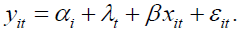The panel data model can be further divided into fixed effect model and random effect model.

Fixed effect model: The individual effect is regarded as a fixed factor that does not change with time, then equation 1 can be expressed as a vector.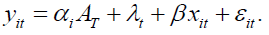In the formula; AT is a column direction where all elements are 1, and the others have the same meaning as the original model.

Random effect model: The individual effect αi is regarded as a random factor that changes with time. By using the random effect model, the long-term factors and short-term factors in the variance can be separated. The basic setting of the model is as follows: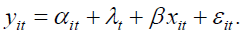Model determination based on Hausman test: Because the missing related variables are not excluded, there will be dependent variable-local GDP will change with the same period correlation of random interference items, and the constraint conditions of exogenous variables are not satisfied, so that the OLS estimator is biased and different. OLS is used to test the fixed effect model and GLS is used to test the random effect model. According to the reference , the difference between the random effect model and the fixed effect model is that it is difficult to try to make a high degree of distinction on the description of individuals. The fixed effect will cost more degrees of freedom, while the random effect is more universal. The proposed Hausman test can be used to distinguish them to some extent (Figure 2).

Advanced random effect model test, test results are stored; Then the fixed effect test is carried out on the model and the results are saved at the same time. Finally, Hausman test is performed on the results to obtain the final model, and the method to verify that both models are satisfied is established in turn.

It can be seen from the output result that the parameter estimation variance of random and fixed effect models under this test is a positive definite matrix, which satisfies the test conditions. Under the 95% confidence interval, the P value is much less than 0.05. Therefore, the fixed effect model should be selected as the explanation model for the influence of economic vitality, while the random effect model should be selected instead.

Model solving process: In this section, stability analysis is conducted on the existing panel data, fixed effect test and random effect test are conducted on the whole data based on the panel mathematical model, and Hausman test is used to determine the applicable model for the panel data. Finally, the analysis results are obtained based on the panel regression model.

Data stability and reliability analysis: The data of this paper comes from China National Statistical Yearbook, which includes the local government's financial expenditure, the total income ofs local international tourism, consumer price index, total profits of enterprises, population, unemployment, tertiary industry, total patents and local GDP. The inconsistency of the order of magnitude of each part will cause trouble to the model fitting. According to the statistical yearbook, the city is divided into 1-31, and the distribution of various data is shown in Figure 3.

Take Figure 3 for example, standardize it first. Assume that the original data is xm, after standardization is Xm.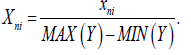After obtaining standardized data, it is shown as follows (Figure 4).

It can be seen from the observation Figure 4 that after the standardization, the feature expression is more clear, which is conducive to the next model inspection work.

Fixed effect test based on OLS: Panel data has the characteristics of separating long-term variables and short-term variables, while fixed effect model focuses on the relationship between variables within the group, It is necessary to test the fixed effect model. The estimation method is OLS estimation, Two assumptions of fixed effect model are made.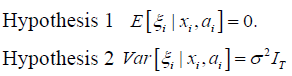The ξ in Hypothesis one is the independent variable interference term.

Hypothesis 1 Assume that the ξ has no effect on the observed value, unobserved value and post observed value.

Hypothesis 2 The general test of homovariance, Ensure that the model satisfies the blue estimate of OLS. And organize data into long data types.

The year (2009-2018) is the cross-section marker, the province (1-31) is the research individual, and each type of independent variable is the influencing factor.

The solution is based on Stata software, and the results are shown in Table 7.

Variables GDP P>|t| [95% Conf. Interval]
Local 0.07766356 0
travel 0.04812193 0.007
index 0.01040376 0.116
profit 0.10857042 0.001
population 0.00840291 0.011
workloser 0.02157164 0.083
third 0.72645815 0
sigma_u 0.03622232 F =0
F(30,272)=29.86
Prob > F = 0.0000
rho 0.91812864
sigma_e 0.01081659

Table 7. Fixed effect test model.

Among them, the F value is very close to 0, indicating that the fixed effect is very significant in this case. Among the seven independent variables, the consumer index and unemployment rate are not significant within the 95% confidence interval.

Local government expenditure, total tourism income, total profits of enterprises, resident population and tertiary industry income all have strong statistical significance. The statistics are shown in Table 8.

Variables GDP
Local 0.0776635
travel 0.0481219
profit 0.10857042
population 0.0084029
third 0.7264581

Table 8. Indicators passing the fixed effect test.

Among them, the third industry has the most significant impact on GDP, and the consumer index has the least impact on GDP.

We can know that all the selected indicators have positive significance for GDP growth within the statistical range. It shows that this test has passed hypothesis one and hypothesis two for panel data, and both of them are true.

Random effect model test based on GLS estimation: The number of indexes (N) is 10, and the time span (T) is 10 years. In this case, it is also possible to meet the random effect model, further test of the random effect model is needed.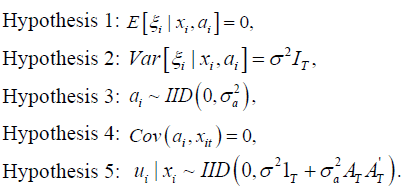According to the above assumption, suppose that the distribution of each independent variable is constrained in a specific case, and the effect of each independent variable obeys the mean value of 0. The second is the description of random interference, which has no correlation with explanatory variables. The third term makes the two coefficients independent of each other.

Based on the above description, GLS estimation method can be used to obtain whether the panel data model conforms to the random effect test when the collected variables are close to the time span.

Organize data into long data types. The year (2009-2018) was used as the cross-section marker, the province (1-31) as the study individual, and each type of independent variable as the influencing factor. Use Stata software to solve the problem, and get the results as shown in Table 9.

Variables GDP P>|t| [95% Conf. Interval]
Local 0.07772621 0
travel | 0.03259963 0.051
index 0.00628094 0.123
profit 0.14530897 0
population 0.00921631 0.332
workloser 0.06423435 0
third 0.71987214 0
sigma_u 0.03622232 F=0.
F(30,272)=29.86
Prob > F = 0.0000
rho 0.91812864
sigma_e 0.01081659

Table 9. Test results of random effect mode.

In 95% confidence interval, P value is 0, that is to say, five hypotheses are passed in this case. This case is suitable for the random effect model (Table 10).

Variables GDP
Local 0.07766353
population 0.00840295
profit 0.14530897
workloser 0.06423435
third 0.71987214

Table 10. Indicators passing the random effect test.

The third industry has the most significant impact on GDP, and the resident population has the least impact on GDP. We can know that all the selected indicators have positive significance for GDP growth within the statistical range. At the same time, it shows that the test has passed all the hypotheses of panel data and satisfies the random effect (Figure 5).

The number of indexes (N) selected in this paper is 10, and the time span (T) is 10 years. In this case, both the fixed effect model and the random effect model are satisfied, and further model test is needed. At this time, Hausman test should be taken.

Model determination based on Hausman test: According to the reference , the difference between the random effect model and the fixed effect model is that it is very difficult to try to distinguish them in a high degree in the description of individuals. The fixed effect will consume a large degree of freedom, while the random effect is more universal on this basis. The proposed Hausman test can be used to distinguish them to some extent.

The test of the advanced random effect model will store the test results, then test the fixed effect of the model and save the result. Hausman test is used to get the final model, and then the method to test the two models simultaneously is established (Table 11).

Variables Value
Chi2(7) 382.02
Prob 0

Table 11. Hausman test results.

It is known from the output that the variance of parameter estimation of random and fixed effect models under this test is a positive definite matrix, which satisfies the test conditions. Under 95% confidence interval, P value is far less than 0.05. Therefore, we should choose fixed effect model as the explanation model of economic vitality.

Analysis of model test results: Using Hausman test, the fixed effect model is determined as the interpretation model of economic vitality, and the results are shown in Table 12.

Variables GDP
Local 0.0776635
travel 0.0481219
profit 0.10857042
population 0.0084029
third 0.7264581

Table 12. Test results of fixed effect model.

Among them, the factors that have a positive impact on economic vitality (GDP) are the local government financial expenditure, the total annual revenue of local tourism, the total annual profit of local enterprises, the local permanent population and the GDP of the tertiary industry.

According to Figure 6, based on the fixed effect model, it can be concluded that the tertiary industry has the largest impact on the estimated vitality, followed by the annual income of enterprises (enterprise vitality), the input expenditure of local government (policy bias), the total income of local tourism, and finally the permanent population. Among them, the influence of the tertiary industry on economic vitality is more than 7 times that of enterprises, indicating that the third vitality can occupy most of the influence among the factors influencing economic vitality.

Activation scheme proposed based on fixed effect model: According to the fixed effect model shown in Figure 4, the explanation degree of each factor to economic vitality has been given, and the following Suggestions are given according to the influence degree.

1. To increase the proportion of the tertiary industry in the overall economy, the tertiary industry plays an important role in the influencing factors, so it is necessary to strengthen the overall proportion of the tertiary industry in the current stage of social construction. Raising the economic proportion of the tertiary industry will greatly promote the improvement of economic vitality.

2. In the process of development, the region should combine its resource endowment and industrial foundation to find the optimal ratio of enterprise structure, complete the adjustment of enterprise structure as soon as possible, and develop appropriate leading industries to promote economic growth. Will be conducive to a steady increase in economic vitality.

3. Local government expenditure has a greater impact on economic vitality. The government needs to be tightly managed to make its spending transparent. We will increase government support for enterprises.

4. Entrepreneurship is encouraged. The government takes the lead in encouraging entrepreneurship, and social practices are carried out to transform enterprises.

According to the influence of individual factors on economic vitality obtained from the fixed model, the influence law of factors is summarized, among which policy adjustment (government expenditure) and enterprise vitality (annual total profit of enterprises) have a greater positive impact on economic vitality, and the implementation of policies in this respect should also be intensified .

The influence of changing trends of population and enterprise vitality on economic vitality: Seven variables were selected, GDP was taken as the expression of economic vitality, and the fixed effect model in the panel data model was used to draw the following conclusions:

The growth rate of permanent resident population has a positive impact on economic vitality, that is, the increase of permanent resident population will increase economic vitality in a small extent.

If the population grows too fast, it will increase the rate of job competition and lead to the rise of unemployment, which will have a negative impact on economic vitality. However, the growth decline of enterprise vitality directly affects the change of economic vitality and presents a positive correlation change.

The establishment of VAR-VCE dynamic volatility model

Based on the panel data in the first question, this section intercepts the local government expenditure of Beijing as a representation of economic policy and establishes a vector autoregressive model (VAR).

Taking economic vitality as the research object, the stability of each factor is verified. Based on vector error correction model (VEC), the lag order and impact function response chart are given to describe the long-term and short-term impact of policy implementation on economic vitality.

Data preparation: Based on the cross-section data of Beijing in the panel data of the whole country, this section conducts relevant test and Analysis on the data, and finally obtains five important factors that have a great impact on the economic vitality: local per capita GDP, local government expenditure, local tourism gross income, local people's living consumption index, and local resident population.

Among them, five kinds of data of Beijing, local per capita GDP, local government expenditure, local tourism gross income, local people's living consumption index, and local resident population are given in Figure 7 after standardization.

It can be seen from Figure 7 that the local government expenditure has a certain increase in each year, basically showing a linear growth; There is no significant change in the local tourism income, which is relatively stable compared with other indicators, indicating that Beijing, as the capital of the country, is very successful in the construction of tourism culture; The resident population gradually declined after reaching the peak from 2012 to 2013, which indicates that Beijing's population has changed greatly and its GDP has grown steadily.

It is not obvious that GDP is affected by the fluctuation of various factors. To understand the dynamic influence of various factors on GDP, we need to carry out vector autoregression for this group of data.

The establishment of vector autoregression (VAR) model: Based on the statistical properties, a function containing the lag value of exogenous and endogenous variables is established to construct the model, which properly explains the influence of the dynamic changes of variables on the dependent variables.

VAR model is essentially a model of multi equation class. Based on the dynamic changes of multiple variables, the interaction between various variables is investigated. Any endogenous variable in the equation system is constructed as the expression of the lag term of any variable. Its general expression is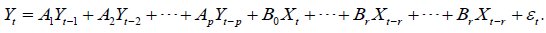Where Yt is the endogenous variable vector of K dimension, Yt-i (i=1,2,…,p) is the vector of lag endogenous variable, Xt-i is the d-dimensional exogenous variable vector or lag exogenous vector. P and R are the lag orders of endogenous and exogenous variables, respectively. Ai is k-order coefficient, Bi is k-row-d-column coefficient matrix, these matrices need to be estimated by specific methods. The last term is a vector composed of k-dimension random error terms. According to the solution of the following figure, we can get the estimation coefficient (Figure 8).

Firstly, the lag order of AVR model is determined according to AIC information criterion and SC criterion when the minimum value is taken, then the lag order is substituted into the meta model, and the coefficient of AVR model can be obtained by OLS estimation.

The establishment of VEC: When multiple time series are unstable, Johansen method is used to test whether there is cointegration relationship. If there is co integration relationship, VEC model can be established to analyze the dynamic relationship of its multi pass model.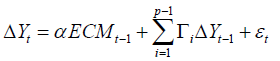In the formula, ECMt-1 is the error correction term. Compared with AVR model, the error correction term is an important feature to distinguish the two.

The error correction term reflects the long-term equilibrium relationship of each variable, and the deviation of long-term equilibrium can be corrected by quick short-term adjustment. Before establishing VEC model, Johansen test is needed to determine the stability and reliability of the model.

Model summary: To determine the regression type of a group of vectors, we need to conduct multiple tests, and finally we can determine whether the model has a correction term. Next, we summarize the model to construct a complete VAR-VEC model.

Solution of the model: In this section, we first judge the stability of time series, and then do ADF test on vector series to judge its stability.

Then, the first-order second-order difference is used to judge its stability. The cointegration test of the original data is carried out, and the satisfied model type is obtained.

Finally, the stability of the model is judged, and the dynamic influence of policy implementation on economic vitality is obtained.

ADF unit root test of vector sequence: First, all the time data are tested by ADF test, and the difference order is 0. The lag order is 1- 2, and the test results are shown in Table 13.

T-Statistic P State
lnGDP -1.679 0.088 Uneven
lnLocal -2.507 0.012 Uneven
lntravel 0.107 0.745 Uneven
lnindex -0.058 0.6614 Uneven
lnpopulation -0.858 0.342 Uneven
lnworkloser -0.402 0.537 Uneven

Table 13. zero order difference ADF unit root test results.

It can be seen from Table 13 that under the time test of order 0 raw data, the t-values of six kinds of t-tests are greater than the comparison data under the confidence interval of 95%, shows that the time series of this group of data do not pass the ADF test of the original data, and further differential test is needed. Carry out difference differentiation on the original data, and continue ADF test on the data after difference, and the results are shown in Table 14.

T-Statistic P state
lnGDP -1.911 0.325 Uneven
lnLocal -2.911 0.049 Uneven
lntravel -1.871 0.345 Uneven
lnindex -1.785 0.359 Uneven
lnpopulation -4.444 0.004 Even
lnworkloser -1.608 0.475 Uneven

Table 14. ADF test results after first-order difference.

It can be seen from Table 14 that under the ADF time series test, the T value of lnpopulation t test in six species is less than the comparison data under the confidence interval of 95%.

Among the six kinds of data, only the population has passed the first-order difference test. The first-order difference of this group of data is not zero, so further difference test is needed.

Carry out the second-order difference differentiation on original data, and continue the ADF test on the data after the difference, and the results are shown in Table 15.

T-Statistic P state
lnGDP -11.634 0 Even
lnLocal -9.1682 0 Even
lntravel -11.72 0 Even
lnindex -11.937 0 Even
lnpopulation -5.342 0 Even
lnworkloser -11.918 0 Even

Table 15. ADF test results after second-order difference.

It can be seen from Table 15 that all the data after the secondorder difference have passed the ADF test, that is to say, this group of data is zero in the second order, and then the inter group cointegration test is carried out.

Figure 9 shows the visual information of three points of each variable under three tests. The confidence intervals of the middle three levels are 1%, 5% and 10% respectively. After the first-order difference, only lnpopulation passed the test; After the second-order difference, all the data pass the test, that is, the group of data is the second-order zero integer data.

Johansen co integration test of variables: According to ADF test, the original variable is a second-order zero integer sequence, that is to say, the original variable is an unstable sequence. First, Johansen co integration test is carried out to find out whether there is a co integration relationship between its combinations.

The test method is to calculate the trace statistics trace and the maximum eigenvalue Max eigevalue. Using the cyclic statistical hypothesis, the existence of cointegration logarithm is assumed. Table 16 shows the Johansen co integration test results.

Hypothesized No. of CE(s) Trace Statistic 0.05 Critical Value Prob.
None 255.6213 95.75366 0
At most 1 161.7542 69.81889 0
At most 2 100.2905 47.85613 0
At most 3 41.5861 29.79707 0.0014
At most 4 7.115546 15.49471 0.5642
At most 5 0.998791 3.841466 0.3176

Table 16. Cointegration test results.

From the trace statistics trace in Table 16, it is assumed that none is the sequence without cointegration.

Under this assumption, the trajectory value is 255.6213, which is greater than the critical value of 95.7537, if the original hypothesis is rejected, there is at least one co integration relationship.

In the case of 5% confidence level, the original assumption is that there are at least four sets of co integration relationship whose trajectory value is less than the critical value, and the determination of the fourth set of co integration relationship is rejected by the assumption.

There are at least three cointegration relations in the linear combination of time series with surface instability.

Establishment and solution of VEC Model: When the original data series are non-stationary time series and Johansen co integration test shows that there are at least three co integration relationships in the series. In order to establish a proper VEC model, it is necessary to determine the optimal lag order of the model. The stability of the model is explained by AR root graph and Roland causality analysis.

Finally, the impulse response chart is given, and the long-term and short-term effects of policy implementation on economic vitality are analyzed.

Determination of lag period based on AIS-SC minimization criterion: When the model is not integrated and stable, multiple VAR models with different lag periods can be established first.

According to the relationship of multiple research variables, the values of each AIC and SC can be recorded and compared.

The optimal lag period of the model can be selected according to the principle of reaching the minimum at the same time. The results in Table 17 are calculated by Eviews software (Figure 10).

D AIC SC
VAR(0) -21.2662 -21.1206
VAR(1) -46.448 -45.4286
VAR(2) -48.0552 -46.16191
VAR(3) -48.7483 -45.98121
VAR(4) -48.81 -44.16917
VAR(5) -48.9 -41.38534
VAR(6) -49.09 -38.70157
VAR(7) -49.1406 -35.87832
VAR(8) -49.2197 -31.0836

Table 17. AIS-SC calculation results.

It can be seen from Table 17 that AIs value decreases with the increase of VAR (N) lag period, presenting a monotonic decreasing state, SC has a minimum at VAR (3). According to AIS information standard and SC standard, the optimal lag time is selected as the third- order lag time.

Determination of VEC model parameters: According to the above analysis, through the co integration test, there are at least three groups of co integration relationships between time series, which can be used to build EVC model.

According to AIS-SC criterion, this model is a third-order lag model, and VAR (3) model should be established. The parameters of the model based on OLS estimation are shown in Table 18.

lngdp lnlocal lntravel lnindex lnpop lnworklose
C 1 -0.7287 -0.76511 1.809693 -0.23384 0.892205
V 0 -0.00682 -0.08337 -0.34303 -0.02693 -0.1565

Table 18. Results of OLS estimation of VEC Model.

Table 18 shows the cointegration formula with the maximum log likelihood.

Thus, the final co integration equation can be written as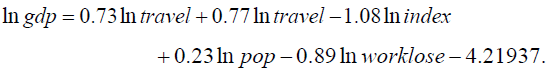Through the co integration relationship, we can see that the long-term equilibrium relationship between economic vitality and local government expenditure, local tourism revenue and local resident population is positive;

There is a long-term negative correlation between economic vitality and local residents' living index and local unemployment rate. According to the test results (see Appendix 1), write the VEC model as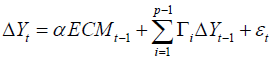The specific coefficients are described as follows: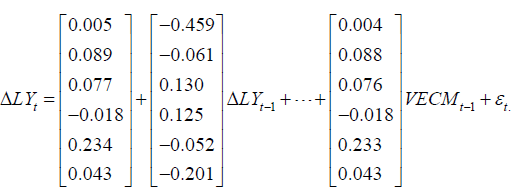In the formula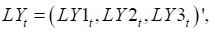the last remainder is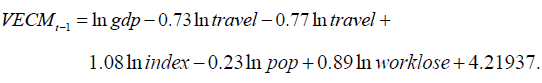Analysis of VEC Model: Before analyzing the model, we need to use the AR root graph method to test the stability of the model, Then, according to the experimental results, the impulse response of VEC model is given, and the long-term and shortterm effects of policy implementation on economic vitality are given under certain circumstances.

Figure 11 is the AR root test. The absolute value of the root is less than one, that is, all the roots are in the plane of the unit circle, and the stability test of the model is passed. The impulse function is applied to the model to observe the long-term and short-term effects of economic policies on economic vitality.

It can be seen from Figure 12 that the promotion effect of economic policies on economic vitality gradually declines after 1-3 periods, and the economic vitality has increased since the third period, Because the experience of implementation after the implementation of economic policies can be applied, which has a secondary effect. After the fourth period, the promoting effect gradually decreased, the decreasing trend was relatively slow, and the long-term positive correlation effect continued.

The model of problem 3

This section aims at question 3. Firstly, we establish a scientific economic vitality index system as the standard of data selection. Secondly, the minimum average difference method is used to screen the data, and the index is initially extracted; Further, the factor analysis method is used to select the main influencing factors, and finally the comprehensive score of each factor is weighted to give the ranking of urban economic vitality.

The construction principle of index system of economic vitality: In order to select effective data to measure the economic vitality of each city, the following five principles are given in this paper, and the general process is as follows (Figure 13):

Scientiic principle: The selection of measurement indicators must be based on scientific principles, and can truly and objectively reflect the impact of various factors on urban economic vitality. The scientific comprehensive index evaluation system of urban economic vitality is the basis of correct analysis and evaluation of regional economic vitality.

Principle of practicability: The construction of evaluation index system is mainly theoretical analysis, which will be affected by the data sources of each index in practical application. Therefore, the availability and reliability of data sources should be ensured in the process of re selecting indicators.

Systematic principle: There should be a certain logical relationship between indicators, which should not only reflect economic vitality from different aspects.

Principle of comparability: The data of each city should conform to comparability, so the data of each city can be compared horizontally and vertically.

Principle of relevance: The comprehensive evaluation index system of regional economic vitality should be an organic combination of a series of related indexes.

Data filtering: The minimum mean square deviation method is used to screen the preliminary data. The observation, value is xij, where i is the number of evaluation objects, i.e. the number of cities, j is the number of evaluation indexes, there are 19 cities, each city has 14 indexes. First, the average value and mean square deviation of index j are calculated.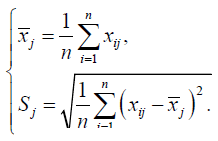Then the minimum mean square deviation of all indexes is calculated, such as: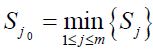If the minimum mean square deviation is close to 0, then the index xj corresponding

Sj can be eliminated and calculated in turn.

Finally, 9 indexes meeting the requirements can be selected from 14 indexes, namely, local GDP, financial expenditure, added value of the primary industry, added value of the tertiary industry, real estate investment, number of college students, population, per capita wage and road traffic noise level.

Factor analysis: Using factor analysis method, the extracted nine indicators, including 190 sample data from 19 cities in 2009-2018, are dimensioned down, and then the coefficient matrix is multiplied by the standardized factor to calculate the score and find out the factors that have the greatest impact on economic vitality.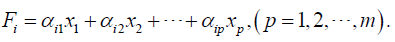Where Fi is the score of the i factor; x1, x2 , xp is the standardized value of the index; the corresponding coefficient is the component score coefficient;

The total factor score is equal to the weighted arithmetic mean of the scores of each factor, that is: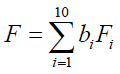Where is the total factor score, Fi is the score of the first influencing factor; Bi is the contribution of the first factor, and factor contribution = variance contribution rate/total variance interpretation after the factor rotation.

Measurement of economic vitality of regional cities: Before measuring the economic vitality of each city, the relationship between variables and factor analysis is further verified through the variance of common factors (Table 19).

Initial Extraction
Local GDP 1 0.943
Financial expenditure 1 0.923
Primary industry 1 0.73
The tertiary industry 1 0.965
Real estate investment 1 0.826
Population size 1 0.908
Per capita wage 1 0.908
Road traffic noise level 1 0.725

Table 19. Common factor variance extraction.

The common factor variance can effectively reflect the strength of its interpretation ability.

The larger the common factor variance extracted between variables, the stronger the ability to be interpreted by the common factor.

Most of the variable factors proposed by the extracted common factor variance are explained to a higher degree than 70%.

Therefore, the extraction effect is better, the information of the original data loss is less, and the data extracted is more reliable.

For the factor whose characteristic root is greater than 1, data analysis is carried out based on SPSS software, and two factors are finally obtained, as shown in the table below, with the explanation of total variance.

From Table 20, it can be seen that the cumulative variance contribution rate is 73.174%, indicating that the first two factors contain 73.174% of all indicator information, and the extracted information is large and highly representative. Therefore, it can be seen that factor analysis is effective in extracting original variable information (Figure 14).

Component Total Cumulative% Cumulative%
1 4.196 46.618 41.315
2 2.39 73.174 73.174
3 0.94 83.622
4 0.859 93.168
5 0.403 97.641
6 0.147 99.271
7 0.048 99.807
8 0.017 100
9 2.13E-16 100

Table 20. Total variance.

It can also be seen from the gravel map that the information contributed by the first two factors in the overall influence factors represents that the broken line is relatively steep, and the slope of the broken line is relatively gentle after that, so it can be considered that the two factors extracted are relatively reasonable (Table 21).

Level 1 2
Local GDP 0.877 -0.418
Financial expenditure 0.837 -0.472
Primary industry 0.338 0.785
The tertiary industry 0.838 -0.511
Real estate investment 0.907 -0.058
College Students 0.328 0.221
Population size 0.7 0.647
Per capita wage 0.7 0.647
Noise level 0.002 -0.475

Table 21. Component matrix.

It can be seen that the primary industry, tertiary industry, college students, population and road traffic noise level are factor 1, which reflects the level of social production and security. Therefore, factor 1 can be named as social production and security factor; local GDP, financial expenditure, real estate investment and per capita wage are factor 2, which reflects the government regulation and control. Therefore, the Factor 2 is called government regulation factor. The contribution rate of factors is analyzed by the method of normal maximization variance, and the conversion correlation coefficient is obtained, which shows the correlation of two factors.

It can be seen from Table 22 that in the component transformation matrix, the value of component one has changed, and the value of component two has also changed. It is necessary to extract the component matrix of the factor load matrix.

Component 1 2
1 0.858 0.514
2 -0.514 0.858

Table 22. Component transformation matrix.

According to the component score coefficient matrix, local GDP, fiscal expenditure, tertiary industry, tertiary industry and real estate investment have a positive impact on the ranking; the primary industry has a negative impact on the ranking. The expression of each influence factor is given according to Table 23.

Name 1 2
Local GDP 0.269 -0.043
Financial expenditure 0.273 -0.067
Primary industry -0.1 0.323
The tertiary industry 0.281 -0.081
Real estate investment 0.198 0.09

Table 23. Component score coefficient matrix.Taking the variance contribution rate of each factor as the weight, the weighted analysis is carried out. After weighted average, the growth index scores are as follows: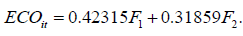The final weight value of each influencing factor is obtained by factor analysis, and the comprehensive score of each factor is obtained by factor score weighting function. The sub factor ranking and comprehensive factor ranking of each city are shown in Table 24.

Ranking Region F1 F2 ECO
1 Shanghai 3.6862 -0.39976 1.395594
2 Beijing 3.29621 -0.30356 1.265118
3 Shenzhen 1.74076 -1.65827 0.190887
4 Guangzhou 1.44806 -0.04957 0.582473
5 Tianjin 0.95879 -0.03063 0.386366
6 Chongqing 0.71899 4.61688 1.767943
7 Wuhan 0.6182 0.05686 0.273524
8 Chengdu 0.41483 0.93521 0.469336
13 Changsha -0.08978 -0.2578 -0.11923
14 Qingdao -0.11201 -0.13905 -0.09058
15 Ningbo -0.2036 -0.56477 -0.26405
16 Dongguan -0.45746 -0.10278 -0.22174
17 Shenyang -0.55388 -0.49867 -0.38771
18 Kunming -0.72876 -0.11422 -0.33748
19 Suzhou -0.8454 -0.05926 -0.36816

Table 24. Score ranking.

It can be seen from the ranking table that the cities such as Beijing, Shanghai and Guangzhou rank the second, third and fourth respectively in the ranking, which indicates that the central economic zone of the country has high stability and is not easy to change. The highest ranking is Chongqing. Shenyang is ranked next, and the transfer of its industrial center may be one of the reasons for this result.

It can be seen from Figure 15 that the ranking of Kunming and Ningbo fluctuates greatly. Considering that the local industrial structure is not obvious enough, it is necessary to strengthen the industrial structure adjustment to improve its economic vitality. Shenyang's ranking is declining year by year, which may also be related to local policies and development strategies, so it needs to be noticed in time.

Comparative analysis of factors affecting economic vitality: In the above, according to the factor analysis method, two main factors that affect economic vitality are social production and security factors and government regulation factors, which have a positive correlation with economic vitality.

According to the 9 influencing factors selected above, the secondary industry, house price, total retail sales of social goods, number of hospitals and number of post offices all have a positive impact on the economic vitality. Comparative analysis is made on each factor to see if there is any difference.

Model establishment: In order to test the accuracy of the index system established to measure economic vitality, considering that the individual effect of each index is not observable and the time effect is not observable, a panel data model is established to test it, and the following model is established: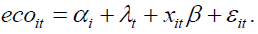In the formula, ecoit is a comprehensive index system to measure economic vitality, xit is an independent variable of N rows and K columns. The factors affecting economic vitality can be divided into

• Social security system: Number of hospitals and Post offices

• Processing and production:The secondary industry

• Consumption level: house price, total retail sales of social goods

Descriptive statistics: In order to analyze the regional economic vitality more specifically, it is necessary to understand the distribution characteristics of each data.

Through descriptive statistical analysis of the data, the basic information of each variable (including sample number, mean value, standard deviation, minimum value and maximum value) is obtained as shown in the Table 25.

Variable N Mean Std.Dev Min Max
ECO 190 0.0378 0.52172 -0.69594 1.76794
The secondary industry 190 4121.713 1882.609 824.59 9732.54
housing price 190 10072.9401 6383.384 3442 47936
Total retail sales 190 4194.3943 2340.761 956.4 12668.7
Number of hospitals 190 402.517 279.872 101 1606
Number of post offices 190 1048.345 1847.484 131 16374

Table 25. Sample description.

In order to analyze the regional economic vitality more specifically, it is necessary to understand the distribution characteristics of each data.

Through descriptive statistical analysis of the data, the basic information of each variable (including sample number, mean value, standard deviation, minimum value and maximum value) is obtained as shown in the Table 25.

It can be seen from Table 25 that the average value of eco is close to 0, indicating that the statistical effect is very good. The fluctuation of house price is large, which is in line with China's national conditions. The number of hospitals is quite different, which deserves the attention of local government. The number of post offices is on the high side in some areas, resulting in waste of resources.

Correlation analysis: Table 25 is the basic situation of the data. After the description and statistics of the data, the correlation analysis of the data is carried out.If the correlation of some indicators is too low, it may lead to the low chi square significance value, which needs to be screened. Then, Pearson correlation coefficient is selected to measure the correlation between the variables.

If the correlation between the explained variables and the explained variables is high, the study of the model is intentional However, if the correlation between explanatory variables is too high, it may lead to collinearity among variables, which may affect the results of the model. The following studies the correlation between the two variables, analyzes the correlation between the two variables and tests its significance.

From the correlation analysis results of Table 26, it can be concluded that the correlation coefficients between all explanatory variables and the interpreted variables are significant, and there is no strong correlation between the explanatory variables.

Correlation ECO The secondary industry Housing price Retail sales Number of hospitals Number of post offices
ECO 1 0.728 0.287 0.842 0.723 0.732
The secondary industry 0.728 1 0.517 0.737 0.346 0.548
Housing price 0.287 0.517 1 0.555 0.151 0.345
Retail sales 0.842 0.737 0.555 1 0.32
Number of hospitals 0.723 0.346 0.151 0.32 1 0.482
Number of post offices 0.732 0.548 0.345 0.661 0.482 1

Table 26. Correlation Analysis.

Therefore, there is no multicollinearity between the explanatory variables. In order to further study the collinearity among the validation variables, the model was validated by using the VIF test, and the results are shown in the Table 27.

t Saliency Tolerance VIF 1/VIF
Constant -36.622 0
Added value of Secondary industry 5.175 0 0.404 2.473 0.404
Housing price -3.072 0 0.513 1.95 0.513
Number of Hospitals 19.921 0 0.581 1.72 0.581

Table 27. VIF test results.

It can be seen from the table that the VIF value of the explanatory variable and the control variable is less than 5, that is to say, the multicollinearity among the variables is low, which will not have a great impact on the results of the model. Therefore, the following modeling and regression analysis can be continued. Continue with the residual analysis (Table 28).

Sum of squares Freedom Mean square F

Saliency
Regression 48.802 5 9,760 679.722 0
Residual 2.642 184 0.014
Total 51.444 189

Table 28. VIF test results.

From the analysis of variance, it can be seen that the F value is far greater than 1, which shows that the differences among the factors are statistically significant, that is, the interaction effect among the factors is more significant. Fixed effect model and mixed model are tested by F test, random effect model and mixed model are tested by BP test, fixed effect model and random effect model are tested by Hausman test, and they are compared (Table 29):

F test BP test Hausman test
Detection value p Detection value p Detection value p
9.46 0 3041.69 0 125.3 0

Table 29. Residual analysis results.

According to the model test results, if the p value corresponding to the F test is 0, less than 0.05, it means that the fixed effect model is due to the mixed model;

If the p value corresponding to the BP test is also 0, less than 0.05, it means that the random effect model is better than the mixed model;

If the p value corresponding to the Hausman test is 0, it means that the fixed effect model tends to be selected. See Table 30 for regression results of fixed effect model to be selected after inspection.

ECO Coef p
F1 0.41315 0
F2 0.31859 0
Added value of secondary industry 0.000659 0
Housing price 0.00893 0.0007
Retail sales of social goods 0.0156 0.001498
Number of hospitals 0.00755 0.135
Number of post offices 0.00149 0.043

Table 30. Fixed effect regression results.

From Table 30, we can see the regression results of the model, and the fixed effect model is selected after the test. The correlation coefficients of the first principal component, the second principal component, the second industry, the house price, the retail sales of social goods, the number of hospitals and the number of post offices are all positively correlated with Eco, indicating that they all have a positive impact on economic vitality. From the perspective of economic vitality, the secondary industry, house price, retail sales of social goods, number of hospitals and number of post offices are all positively related to economic vitality. In these variables, when one variable changes, the other variables remain unchanged, then the economic vitality changes in the same direction. Therefore, it can be further proved that the economic vitality index system constructed in this paper can accurately measure the economic vitality.

## A development plan based on the perspective of the decision maker

This section first reviews questions 1 to 3 above, and obtains the general universality of the model established in this paper. Finally, according to the results, it proposes measures conducive to improving economic vitality and promoting economic development.

Conclusion review

From question 1 to question 3, we can roughly divide the index system of urban economic vitality into indicators of economic growth, indicators of attractiveness to capital and production factors, indicators of employment and residents' quality of life, indicators of innovation capacity and indicators of intellectual property protection.

Which chose the per capita GDP, fiscal revenue, education and human capital, income levels, employment, innovation and intellectual property rights protection for data collection, processing, modeling and analysis, and it can analysis indicators and economic vitality all remain positive correlation, therefore, we can analysis from the perspective of the above and advise the sustainable development of the economic vitality of benign and stronger regional competitiveness.

Suggestions on the benign sustainable development of Beijing's economic vitality

Economic vitality includes not only the speed, stability and results of economic growth, but also the average quality of life of the people, such as the level of education and health standards, as well as the overall progress of the economic structure and social structure (Figure 16).

In view of the excessive factors affecting economic vitality, we roughly divide the policy into three parts, including optimizing industrial structure (O), rationally controlling investment intensity (R) and technological innovation (T), namely ORT development strategy.

Optimizing the industrial structure

Vigorously developing the tertiary industry: The third industry is an important indicator of a country's economic development. And the tertiary industry has the characteristics of less investment, short cycle, quick effect and high wages of employees. Vigorously developing the tertiary industry can rapidly expand employment fields and jobs, avoid labor surplus, and improve residents' income. For modern cities, residents not only have material needs, but also pursue spiritual level. This development trend promotes the region to continuously develop new industries to meet the needs of the people, so as to improve residents and to improve the quality of life. Therefore, we vigorously develop the tertiary industry, which has a significant role in promoting the sustainable development of economic vitality.

Strengthening the development of primary and secondary industries: For the adjustment of Beijing's economic structure and the promotion of its regional competitiveness, it is necessary to develop the tertiary industry while strengthening the primary industry and expanding the scale of the secondary industry. The first industry is the basic industry of the national economy, strengthening the first industry, and laying the foundation for the development of the second industry and the third industry.

Reasonable control of investment: Investment is an important part of GDP, but also an element of economic vitality. The growth of investment is of great significance to the promotion of economic vitality. In China, investment is mainly divided into private investment, government investment and foreign investment. From the perspective of Beijing, as a first-tier city, a large number of foreign enterprises and state-owned enterprises invest in Beijing in various forms. However, unreasonable investment may cause the princess of resources, leading to the imbalance of social and economic development. Therefore, Beijing should control the scope of investment and improve the efficiency of investment.

Technology innovation: Science and technology are the primary productive forces, and innovation is a force that cannot be ignored to drive economic development. Innovation is conducive to the optimization and transformation of China's economic growth mode. Economic vitality comes from the sound growth of economy.

We should strengthen the policy support for the investment in science and technology of enterprises, guide the flow of resources such as projects, funds and talents to enterprises, and establish an innovation support system with enterprises as the main body. Cultivate and develop the next generation Internet, new generation mobile communication, Internet of things, navigation and location services, biomedicine and other hightech strategic emerging industries. Accelerate the construction of high-end talents gathering special zone, and actively introduce and cultivate high-end talents.

## Model Evaluations

The advantages and disadvantages of model factor analysis and panel data model are analyzed.

Factor analysis: Through dimensionality reduction of a variety of impact indicators, the main factors are extracted from the complex factors, and a few factors are used to describe the relationship between many indicators, that is, several closely related indicators are classified into the same category, each category of indicators becomes a factor, and the economic vitality is measured by a few factors. It simplifies the problem, measures most of the information of economic vitality, and gets more scientific and accurate information at the same time.

Panel data model: Compared with the traditional time series model, the panel data model can provide more data points, increase the degree of freedom of data and reduce the degree of collinearity between explanatory variables, thus improving the effectiveness and accuracy of model estimation; Panel data model is more conducive to reflect the randomness of the gap between individuals. In this paper, panel data model can not only reflect the information between the given factors, but also reflect the information of a certain factor through the study of other influencing factors.

Improvements needed

When using panel data model to study influence factors, there are some difficulties in variable design and data collection, some errors in factor prediction, and selection difficulties in influence factors; panel data analysis of time series of factors is short, which can only reflect the data characteristics in the short term, not the long-term changes of factors.

## Conclusion

Promotion and application of the model

In this paper, three models are established: Panel Data Model (fixed effect model and random effect model), Avr-Ave model, and principal component index system model. The Var-Vec model has great generalization. Applicable fields are:

Research on agricultural economic development based on var. Because of its unique ability to test the dynamic fluctuation, AVR can solve the research difficulties in the agricultural economy which are greatly affected by the quarterly seasonality.

Oil price evaluation, international oil has been afected by many aspects, among which the fluctuation of international political content has a great impact. The VAR model can highlight the impact of oil price changes in the short term and make adjustments at any time.

Get the App